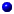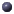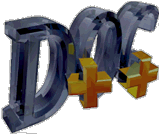## Inheritance:

### Public Methodsvirtual coordinate coord()PolarSatPosition* next()int plane()PolarSatPosition(double = 1000, double = 90, double = 0, double = 0, double = 0)void set(double Altitude, double Lon, double Alpha, double inclination=90)

### Protected Fieldsdouble inclination_PolarSatPosition* next_
Next intraplane satelliteint plane_
Orbital plane that this satellite resides in

### Protected Methodsint command(int argc, const char*const* argv)

### Public Fieldsstatic double time_advance_

### Public MethodsNode* node()int type()

### Protected Fieldscoordinate initial_Node* node_int type_

## DocumentationPolarSatPosition(double = 1000, double = 90, double = 0, double = 0, double = 0)virtual coordinate coord()void set(double Altitude, double Lon, double Alpha, double inclination=90)PolarSatPosition* next()int plane()int command(int argc, const char*const* argv)PolarSatPosition* next_
Next intraplane satelliteint plane_
Orbital plane that this satellite resides indouble inclination_